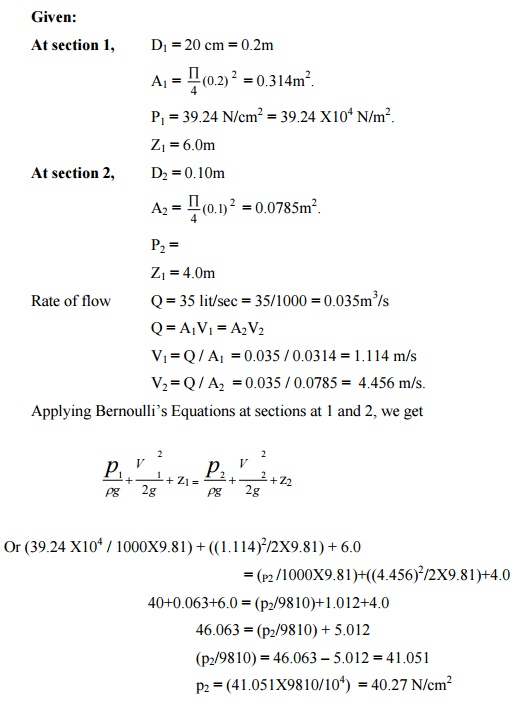# The water is flowing through a pipe having diameters 20 cm and 10 cm at sections 1 and 2 respectively. The rate of flow through pipe is 35 lit/sec. the section 1 is 6m above datum. If the pressure at section 2 is 4m above the datum. If the pressure at section 1 is 39.24 N/cm2, find the intensity of pressure at section 2.

Question-AnswerCategory: Fluid MechanicsThe water is flowing through a pipe having diameters 20 cm and 10 cm at sections 1 and 2 respectively. The rate of flow through pipe is 35 lit/sec. the section 1 is 6m above datum. If the pressure at section 2 is 4m above the datum. If the pressure at section 1 is 39.24 N/cm2, find the intensity of pressure at section 2.

The water is flowing through a pipe having diameters 20 cm and 10 cm at sections 1 and 2 respectively. The rate of flow through pipe is 35 lit/sec. the section 1 is 6m above datum. If the pressure at section 2 is 4m above the datum. If the pressure at section 1 is 39.24 N/cm2, find the intensity of pressure at section 2.